# Quasi-periodic motion

A motion of a particle according to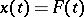, where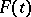is a (vector-valued) quasi-periodic function.

Let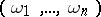bereal numbers,, that are incommensurate, i.e. independent over, i.e.,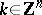, implies. Then the "rectilinear motion" on the torus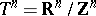defined byis quasi-periodic (and not periodic because of the incommensurability). This is typical in the sense that any quasi-periodic motion is a composition of this with a vector-valued periodic function.

Let a Hamiltonian function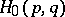define a completely-integrable system (cf. Completely-integrable differential equation). Then there are new symplectic coordinates, called action angle coordinates,in which the Hamiltonian takes the form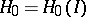and the Hamiltonian equations become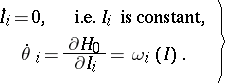(a1)

The phase space in this case fibres into invariant tori of dimension, and the motion on each is of the form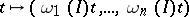i.e. quasi-periodic (or periodic, or a mixture of these two, Arnol'd's theorem). (In [a1] these motions are called conditionally periodic.) These tori are the level sets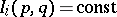,. Such a torus is called a non-resonant torus if the corresponding frequencies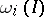are functionally independent, i.e. if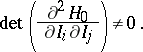(a2)

It can be shown that the non-resonant tori form a set of full measure. Nevertheless, invariant resonant tori do exist and they are mixed in such a way that they too form a dense set.

Now consider a small perturbation of an integrable system satisfying (a2):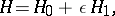whereis periodic in the angle variables. The famous KAM theorem (Kolmogorov–Arnol'd–Moser theorem) says that ifis small enough, then for almost-all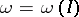there exists an invariant torus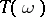of the perturbed system that is close to the invariant torus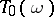of the unperturbed system. The theorem was stated (in an analytic case) by A.N. Kolmogorov [a2] and proved by V.I. Arnol'd and J. Moser (different cases) [a3], [a4]. Moreover, the union of the invariants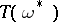forms a set of positive measure and the measure of the complement goes to zero as. There is much more to this theorem. In particular, it specifies a set ofwhich do indeed have invariant tori associated to them. These arewhich are "sufficiently incommensurate" , which means that they satisfy an equality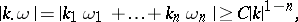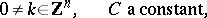where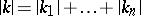. There are "many" such, enough for the theorem as stated. It is also true that some such condition is necessary. For example, ifand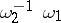is rational, then there are arbitrarily-small perturbations which will destroy the torus(a fact already known to H. Poincaré). There are many other aspects to KAM theory, for instance KAM-type theorems for the case of equilibrium points and for systems with additional time-periodic forces acting on them, cf. e.g. [a5], [a1]. There are also converse KAM-theorems, which give conditions on a region of space which guarantee that there are no invariant tori, [a11], [a12]. Some of the invariant KAM tori of the system break up (asgrows) into Cantor set like sets; these are in this context sometimes known as Cantori.

If, the iso-energy surfaces are of dimension 3, the invariant KAM tori in them are of dimension 2 and these hence separate the energy surfaces into disjoint pieces. As a result the orbits which are not on the invariant tori are trapped between them. If the frequencies of the tori depend differentiably on the values of the action variables defining it, which is guaranteed by the iso-energy non-degeneracy condition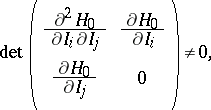then it follows that the action variables for all initial values remain near there and exhibit no secular perturbation phenomena. For more than 2 degrees of freedom the invariant tori do not separate the iso-energy surfaces into disjoint pieces and it is indeed possible, also in the iso-energy non-degenerate case, that the action variables drift away from their initial values. This phenomenon is known as Arnol'd diffusion.

An example of an integrable system is the planetary system with the interactions between the planets disregarded; adding this interaction gives the small perturbation.

How to Cite This Entry:
Quasi-periodic motion. Encyclopedia of Mathematics. URL: http://encyclopediaofmath.org/index.php?title=Quasi-periodic_motion&oldid=49383